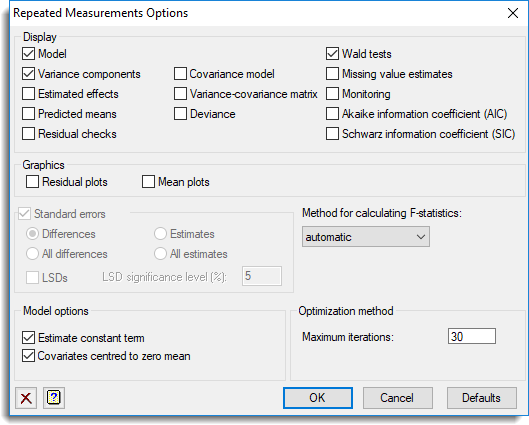1. Home
2. Repeated Measures (Data in parallel) Options

# Repeated Measures (Data in parallel) Options

Selects information to be printed by the analysis and controls certain aspects of the method used.## Display

This specifies which items of output are to be produced by the analysis.

 Model Description of the model fitted by the analysis Variance components Estimates of variance parameters Estimated effects Estimates of regression coefficients Predicted means Predicted means Residual checks Uses the VCHECK procedure to check the residuals for outliers and variance stability Stratum variances Estimates of approximate stratum variances Covariance model Estimated covariance models in matrix format Variance-covariance matrix Variance-covariance matrix for the variance parameters Deviance The residual deviance Wald tests Wald tests for fixed model terms and accompanying F-statistics (if selected) Missing value estimates Estimates of values missing from the input Monitoring Monitoring information at each iteration Akaike information coefficient (AIC) Akaike information coefficient to assess the random model Schwarz information coefficient (SIC) Schwarz information coefficient to assess the random model

## Standard errors

Tables of means and effects are accompanied by estimates of standard errors. You can choose whether Genstat computes standard errors or standard errors of differences (SEDs) for the tables.

## Method for calculating F-statistics

This controls whether Wald tests for fixed effects are accompanied with approximate F statistics and corresponding numbers of residual degrees of freedom. The computations, using the method devised by Kenward & Roger (1997), can be time consuming with large or complicated models. So, the default setting automatic, can be used to allow Genstat to assess the model itself and decide automatically whether to do the computations and which method to use. The other settings allow you to control what to do yourself:

 none No F statistics are produced algebraic F statistics are calculated using algebraic derivatives (which may involve large matrix calculations) numerical F statistics are calculated using numerical derivatives (which require an extra evaluation of the mixed model equations for every variance parameter).

## Estimate constant term

Specifies whether a constant term is included in the fixed model.

## Covariates centred to zero mean

Specifies whether covariates are centred to zero mean during the analysis. This applies to all covariates in the model. If covariates are centred, tables of predicted means are based on the mean covariate value, otherwise zero for each covariate.

## Maximum iterations

This specifies the maximum number of iterations to use to optimize the REML likelihood.